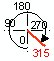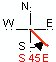Go to ET GeoWizards 12.x User Guide

COGO Inverse

Go to ToolBox Implementation

Converts a polyline or a polygon data set to a feature class containing only single segmented polylines. For each segment the COGO attributes are calculated and added to the resulting attribute table. The attributes of the original features are copied to the output features.

Inputs:

• A Polyline, PolylineZ(M), Polygon, PolygonZ(M)  feature layer

Options:

• Direction Angle Type - the type of the output angle for the direction of the segments
 Polar North Azimuth South Azimuth Quadrant Bearing• Direction Angle Units
• DD - Decimal Degrees
• DMS - Degrees Minutes Seconds
• Gon - Gons - used in some European countries (One gon is equal to 1/400 circle)
• Precision
• Linear - integer indicating the number of places after the decimal point for the output linear measurements
• Angular - integer indicating the number of places after the decimal point for the output angular measurements

Outputs:

• New polyline feature class
• Attribute fields added to the attribute table of the output feature class
• Always
• [Direction] - the direction of the segment. The angle can be measured in arithmetic or geographic notation depending on the user choice
• [Distance] - the length of the segment measured in the units of the original dataset
• [Delta] - for circular arcs only. The central angle of the circular arc in degrees
• [Radius] - for circular arcs only. The radius of the circular arc
• [Tangent] - for circular arcs only. The distance from the Start/End points of the circular arc to the intersection point of the tangents
• [ArcLength] - for circular arcs only. The length of the circular arc
• [Delta] - for circular arcs only. The central angle of the circular arc
• [Side] - the side of the circular arc compared with the tangent in the start point
• User choice
• [XStart] - X coordinate of the start point of the segment
• [YStart] - Y coordinate of the start point of the segment
• [XEnd] - X coordinate of the end point of the segment
• [YEnd] -Y coordinate of the end point of the segment
• User choice - shapes with Z values only
• [ZStart] - Z coordinate of the start point of the segment
• [ZEnd] - Z coordinate of the end point of the segment
• [Slope] - the slope of the segment in degrees (from -90 to 90)
• User choice - shapes with M values only
• [MStart] - M coordinate of the start point of the segment
• [MEnd] - M coordinate of the end point of the segment

Notes :

• The Z or M fields will be added only if the source dataset  has Z/M values
• Since the feature class format cannot handle true arcs, if the source dataset is Geodatabase (Personal or SDE) and contains true arcs, these arcs will be represented by their linear approximation. One record will be created for each arc and all the COGO attributes of the original arc will be calculated

Command line syntax

Parameters

Expression Explanation
<input_dataset> A Polyline or Polygon feature class or feature layer
<out_feature class> A String - the full name of the output feature class (A feature class with the same full name should not exist)
<NorthAzimuth | SouthAzimuth | Polar | QuadrantBearing> Direction Type. A String -  the type of the output angle for the direction of the segments.
<DD | DMS | Radians | Gradians | Gons> Direction Unit. A String -  the type of the output angle for the direction of the segments.
{linear_precision} A Number  representing the number of places after the decimal point for the output linear measurements
{angular_precision}

A Number  representing the number of places after the decimal point for the output angular  measurements

{add_start_end_coordinates} A Boolean  indicating whether the Start & End point coordinates will be recorded for the segments
{add_ZM_attributes} A Boolean  indicating whether the Z/M values of the segments will be recorded in the output attribute table. If the input features do not have Z/M values this parameter is set to False automatically

Scripting syntax